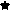### Research

I am interested in the study of symmetry in the context of noncommutative algebras. My research includes interactions with noncommutative algebraic geometry, Hopf algebras and quantum groups, mathematical physics, and representation theory.

### Survey articles

Items below marked with aindicate collaborations with a Jeopardy! champion.

### Student Research

• Thomas Lamkin, Thy Nguyen, and Caleb Wright: Four-vertex quivers supporting twisted graded Calabi-Yau algebras
(preprint)

Abstract: We study quivers supporting twisted graded Calabi-Yau algebras, building on work of Rogalski and the first author. Specifically, we classify quivers on four vertices in which the Nakayama automorphism acts on the degree zero part by either a four-cycle, a three-cycle, or two two-cycles. In order to realize algebras associated to some of these quivers, we show that graded twists of a twisted graded Calabi-Yau algebra is another algebra of the same type.

• Thomas Lamkin: Centers and automorphisms of PI quantum matrix algebras
To appear in AMS Contemporary Mathematics, "Geometric and Algebraic Aspects of Quantum Groups and Related Topics".
(preprint, poster)

Abstract: We determine a presentation of the center of the quantum matrix algebras for q a nontrivial odd root of unity, and discuss its application in studying the automorphism group of Mq(2). We also show that the automorphism group of Mq(n), for all n≥2, contains a free group on two generators.

• Jacob Barahona Kamsvaag: Auslander's Theorem for dihedral actions on preprojective algebras of type A
Canadian Mathematical Bulletin, 2023, 66 (1), 324-339.
(preprint, slides)

Abstract: Given an algebra R and G a finite group of automorphisms of R, there is a natural map ηR,G:R#G → EndRG(R), called the Auslander map. A theorem of Auslander shows that ηR,G is an isomorphism when R=C[V] and G is a finite group acting linearly and without reflections on the finite-dimensional vector space V. The work of Mori and Bao-He-Zhang has encouraged study of this theorem in the context of Artin-Schelter regular algebras. We initiate a study of Auslander's result in the setting of non-connected graded Calbi-Yau algebras. When R is a preprojective algebra of type A and G is a finite subgroup of Dn acting on R by automorphism, our main result shows that ηR,G is an isomorphism if and only if G does not contain all of the reflections through a vertex.

• Thomas Lamkin: Universal Enveloping Algebras of Poisson Superalgebras
(preprint, poster)

Abstract: In this paper, we define and study the universal enveloping algebra of Poisson superalgebras. In particular, a new PBW theorem for Lie-Rinehart superalgebras is proved, leading to a PBW theorem for Poisson superalgebras; we show the universal enveloping algebra of a Poisson Hopf superalgebra (resp. Poisson-Ore extension) is a Hopf superalgebra (resp. iterated Ore extension); and we determine the universal enveloping algebra for examples such as quadratic polynomial Poisson superalgebras and Poisson symmetric superalgebras.

• Phuong Ho: Fixed rings of quantum generalized Weyl algebras
Communications in Algebra, 2020, 48 (9), 4051-4064
(preprint, slides, poster)

Abstract: Generalized Weyl Algebras (GWAs) appear in diverse areas of mathematics including mathematical physics, noncommutative algebra, and representation theory. We study the invariants of quantum GWAs under finite automorphisms. We extend a theorem of Jordan and Wells and apply it to determine the fixed ring of quantum GWAs under diagonal automorphisms. We further study properties of the fixed rings, including global dimension, rigidity, and simplicity.

• Delaney Aydel: Classifying actions of Tn ⊗ Tn on Path algebras of quivers
(slides)

Abstract: Let Tn denote the nth Taft algebra. We classify actions of T2 ⊗ T2 as extensions of actions of Z2 x Z2 on path algebras of four-vertex Schurian quivers. These actions are required to be inner faithful. An example is computed in full, and results are given briefly for the remaining types of actions.

• Siqi Li: Stabilizers of canonical matrices arising from matrix congruence
(poster)

Abstract: My research is in noncommutative algebra and my project was to study the stabilizer groups of special matrices arising in the theory of congruent matrices as well as their applications. Our goal was to determine canonical forms under a certain type of congruence which corresponds to noncommutative quadratic polynomials in degree three. We extended results in the 2x2 case by Gaddis to the case of 3x3 matrices. Canonical forms under standard matrix congruence were developed by Horn-Sergeichuk. We first listed their 3x3 forms, and then computed the stabilizer groups for a representative of each form. Next, we proceeded to determine the canonical forms under standard form congruence for 4x4 matrices. This involved developing routines in Maple to aid in the computation. Additionally, we developed some general rules and conjectures for stabilizer groups.

• Ke Liang: Four-Vertex quivers supporting graded Calabi-Yau algebras
(poster)

Abstract: Our project focused on specific shapes arising from string theory, called Calabi-Yau manifolds, and the algebraic objects associated to them. The goal is to classify directed graphs (called quivers) on four vertices supporting graded Calabi-Yau algebras of global dimension 3 and finite GK dimension. The bulk of our study was to analyze the matrix-valued Hilbert series associated to each quiver. During our study, we found many `good' matrices which support Calabi-Yau algebras, including certain circulant matrices, as well as matrices associated to McKay quivers and mutations. We also ruled out large classes of matrices which cannot support Calabi-Yau algebras. This extends work of Gaddis, Reyes, and Rogalski.